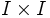# Three sides lemma

(diff) ← Older revision | Latest revision (diff) | Newer revision → (diff)

This term is nonstandard and is being used locally within the wiki. For its use outside the wiki, please define the term when using it.

## Statement

The inclusion of three sides in the unit square is equivalent to the inclusion of one side in the unit square, viz., is equivalent to the inclusion of a boundary$I$ in$I \times I$.

An explicit isotopy can also be written down, which is useful in some situations.

## Applications

• The three sides lemma is sometimes used to give a different definition of the second homotopy group of a pair. In fact, the generalized three sides lemma is used to give alternative definitions for all the higher homotopy groups.
• The three sides lemma gives a quick proof that the inclusion of the two endpoints in the unit interval is a cofibration (contrast this with the two sides lemma, which shows that the inclusion of one endpoint is a cofibration). An explicit retraction can also be written down.
• The three sides lemma gives a proof that the inclusion of the disjoint union of the center and boundary sphere of a disc, in the disc, is a cofibration. This is used to prove that the inclusion of a point in a manifold is a cofibration Further information: Center plus boundary in disc is cofibration